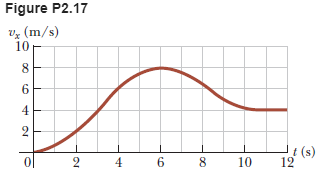# Problem: Figure P2.17 shows a graph of vx versus t for the motion of a motorcyclist as he starts from rest and moves along the road in a straight line. (a) Find the average acceleration for the time interval t = 0 to t = 6.00 s. (b) Estimate the time at which the acceleration has its greatest positive value and the value of the acceleration at that instant.(c) When is the acceleration zero? (d) Estimate the maximum negative value of the acceleration and the time at which it occurs.

###### FREE Expert Solution

Acceleration is expressed as:

$\overline{){\mathbf{a}}{\mathbf{=}}\frac{\mathbf{∆}\mathbf{v}}{\mathbf{∆}\mathbf{t}}}$, where Δv is the change in velocity and Δt is the time interval over this change.

Δv = vf - vi

Similarly, Δt = tf - ti

So that we now have:

$\overline{){\mathbf{a}}{\mathbf{=}}\frac{{\mathbf{v}}_{\mathbf{f}}\mathbf{-}{\mathbf{v}}_{\mathbf{i}}}{{\mathbf{t}}_{\mathbf{f}}\mathbf{-}{\mathbf{t}}_{\mathbf{i}}}}$

(a)

The acceleration of the velocity-time graph is the slope of the graph.

The average acceleration for the time interval t = 0 to t = 6.00 s is:

92% (224 ratings)###### Problem Details

Figure P2.17 shows a graph of vx versus t for the motion of a motorcyclist as he starts from rest and moves along the road in a straight line.(a) Find the average acceleration for the time interval t = 0 to t = 6.00 s.

(b) Estimate the time at which the acceleration has its greatest positive value and the value of the acceleration at that instant.

(c) When is the acceleration zero?

(d) Estimate the maximum negative value of the acceleration and the time at which it occurs.

Frequently Asked Questions

What scientific concept do you need to know in order to solve this problem?

Our tutors have indicated that to solve this problem you will need to apply the Velocity-Time Graphs & Acceleration concept. You can view video lessons to learn Velocity-Time Graphs & Acceleration. Or if you need more Velocity-Time Graphs & Acceleration practice, you can also practice Velocity-Time Graphs & Acceleration practice problems.

What professor is this problem relevant for?

Based on our data, we think this problem is relevant for Professor McCormick's class at USF.